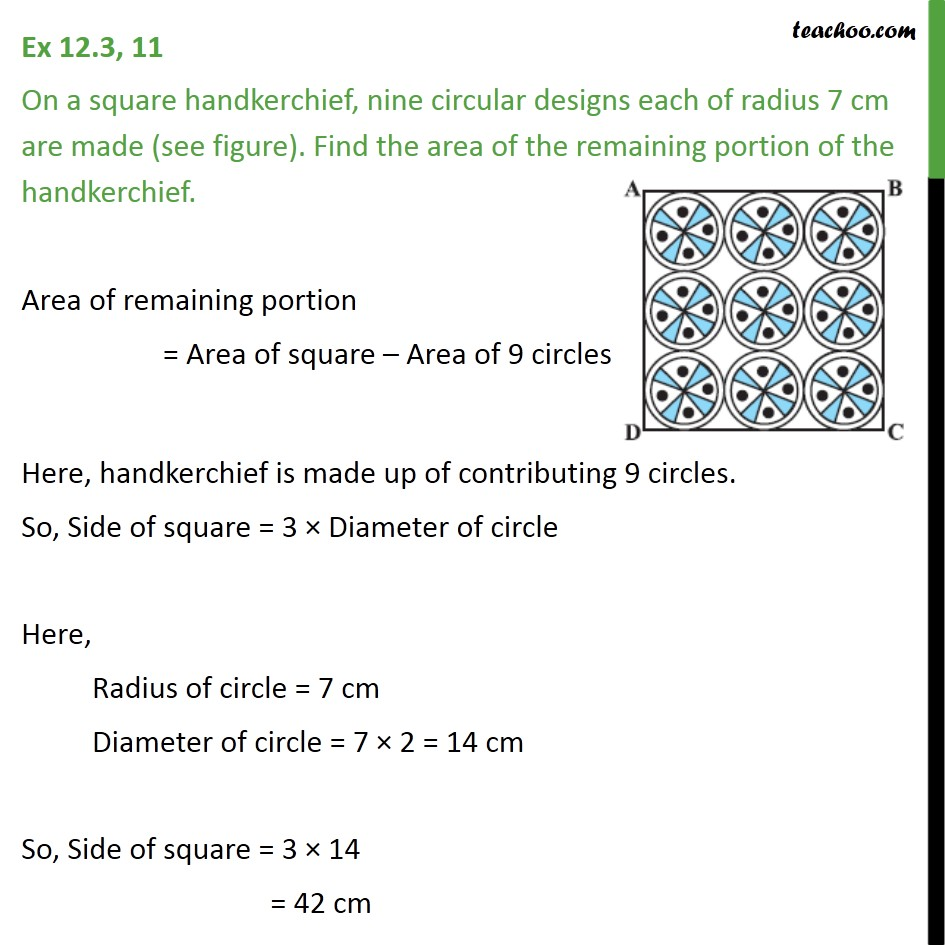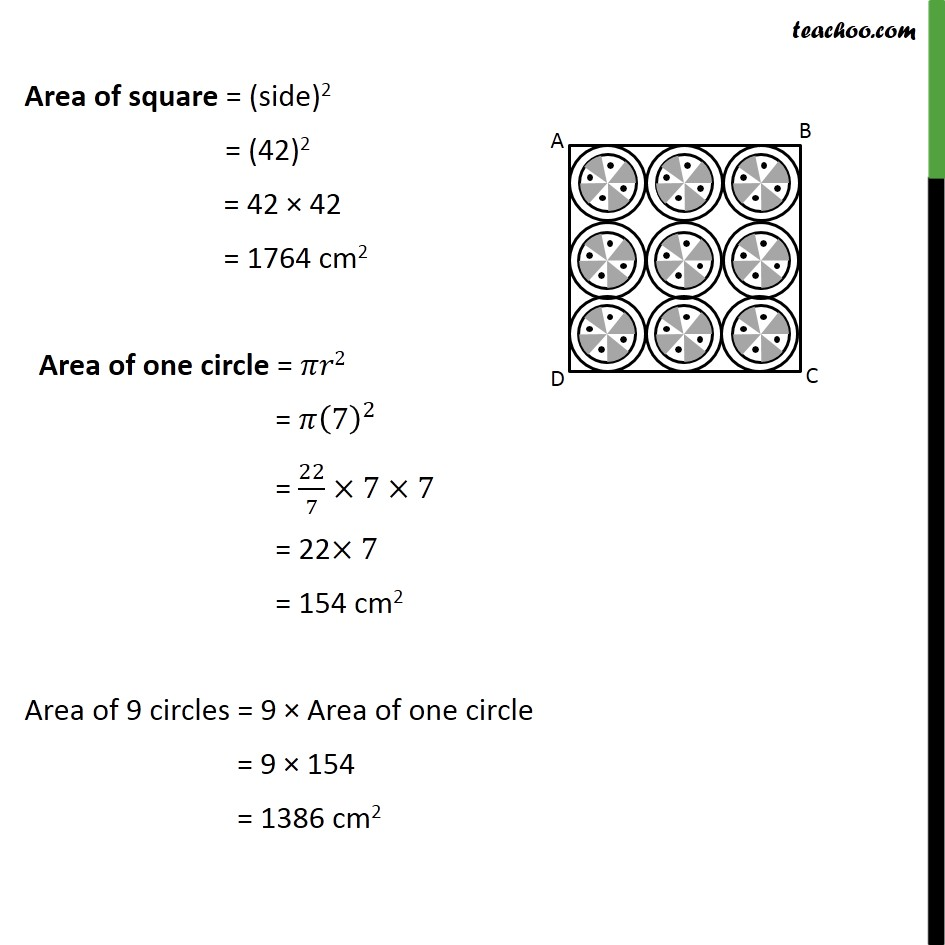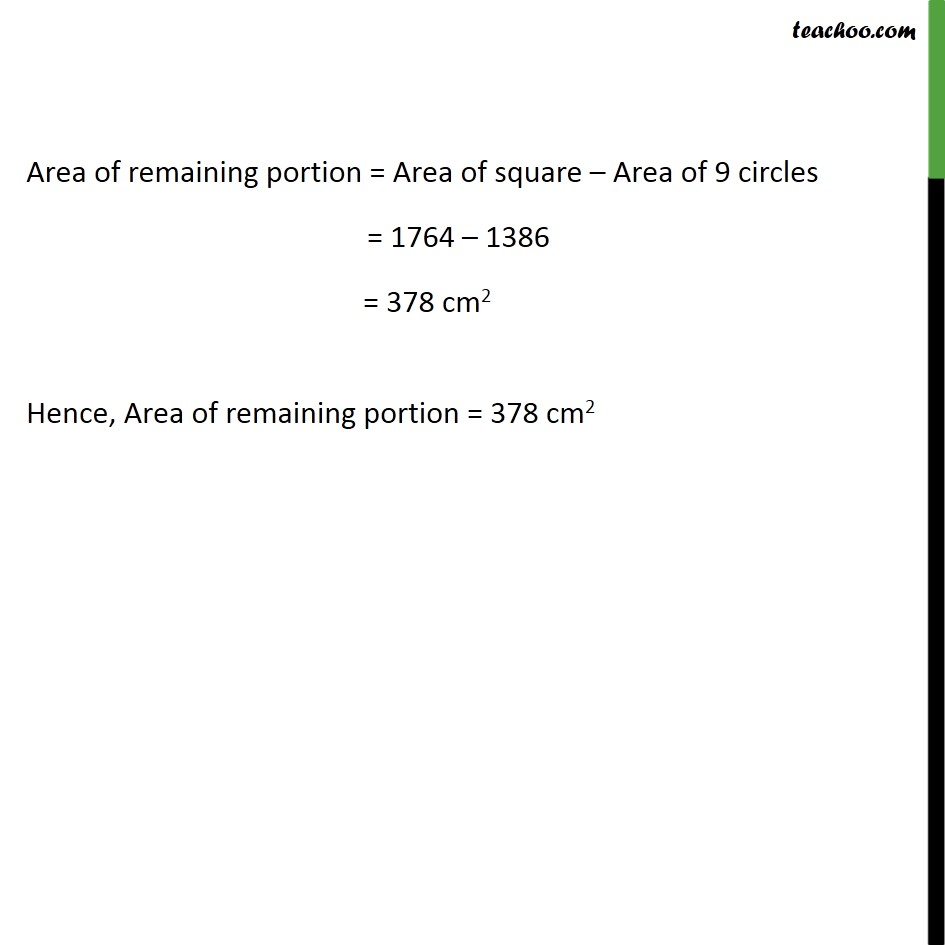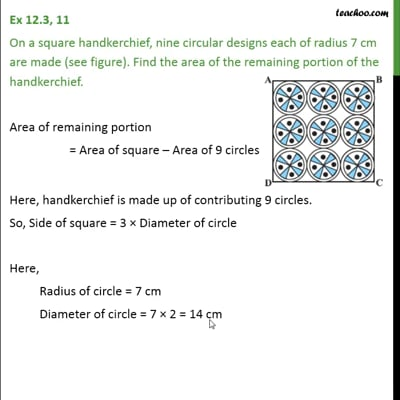Area of combination of figures : circle based

Chapter 12 Class 10 Areas related to Circles
Concept wiseThis video is only available for Teachoo black users

Solve all your doubts with Teachoo Black (new monthly pack available now!)

### Transcript

Ex 12.3, 11 On a square handkerchief, nine circular designs each of radius 7 cm are made (see figure). Find the area of the remaining portion of the handkerchief. Area of remaining portion = Area of square Area of 9 circles Here, handkerchief is made up of contributing 9 circles. So, Side of square = 3 Diameter of circle Here, Radius of circle = 7 cm Diameter of circle = 7 2 = 14 cm So, Side of square = 3 14 = 42 cm Area of square = (side)2 = (42)2 = 42 42 = 1764 cm2 Area of one circle = 2 = (7)^2 = 22/7 7 7 = 22 7 = 154 cm2 Area of 9 circles = 9 Area of one circle = 9 154 = 1386 cm2 Area of remaining portion = Area of square Area of 9 circles = 1764 1386 = 378 cm2 Hence, Area of remaining portion = 378 cm2